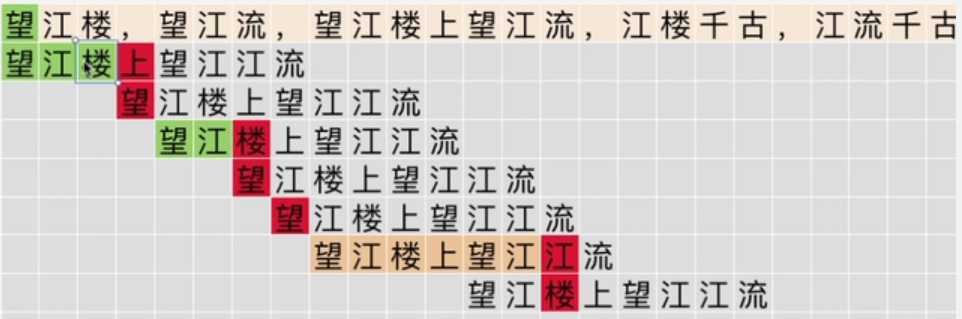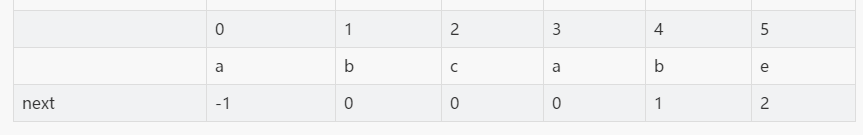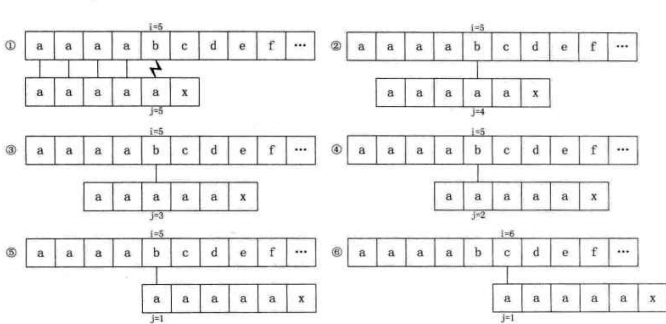# KMP算法

BF算法（暴力匹配算法）

#include<bits/stdc++.h>
using namespace std;
int main() {
char s, p;
scanf("%s %s", s, p);
int i = 0, j = 0;
int len1 = strlen(s);
int len2 = strlen(p);
while(i < len1 && j < len2) {
if(s[i] == p[j]) {//当文本串和模式串字符相匹配时，让文本串下一个元素和模式串下一个元素继续比较
i++;
j++;
}
else { //如果发生了不匹配，则模式串返回第一个字符，文本串回到与模式串首元素对应的位置的后一个位置，即c-d+1位置
i = i - (j - 1);
j = 0;
}
}
if(j == len2)printf("找到匹配字符串，位于文本串第%d个字符位置 ", i - j + 1);
elseprintf("未找到匹配字符串 ");
}

KMP算法
KMP算法实现（1）如果文本串的第0， 1， 2个字符与模式串的第0， 1， 2个字符匹配上，第3个字符匹配失败，按照BF算法的思路应该让文本串的”江“字与模式串的”望“字进行比较，而在第一次循环的比较中我们已经得知”江楼“相匹配，如果再做重复的比较就没有意义了，所以我们使得i位置不变，令P串的j回溯到第0个字符”望“。此时i=3, j=0。
（2）进行下一步比较，此时文本串i位置的字符与模式串j=0的字符不匹配，此时需要使得文本串i的下一个字符和模式串的这个字符相比较，匹配失败。此时i=4,j=0。
（3）进行下一步比较，文本串的”望江“和模式串的”望江“相匹配，”楼“字不匹配，使得i不变，j回溯到模式串的第0个字符。此时i=6,j=0。
（4）i=6,j=0处的字符不匹配，使得文本串i的下一个字符和模式串的这个字符相比较，匹配失败。此时i=7，j=0。
（5）i=7,j=0处的字符不匹配，使得文本串i的下一个字符和模式串的这个字符相比较，匹配失败。此时i=8，j=0。
（6）文本串的i=8位置的字符到i=13位置的字符与模式串的j=0位置的字符到第j=5位置的字符相匹配，即图中橙色部分的比较，此时i=14,j=6。
（7）i=14,j=6处的字符不匹配，i不变，j回溯，按照（1）的思路，应使得j回溯到0位置。但是由于模式串的第二个望江与第一个望江是一样的字符，而第二个望江与文本串的望江又相匹配，因此再次比较第一个望江和文本串中的望江是毫无意义的，因此使j回溯到模式串第一个望江后面的位置，即j=3。此时i=14,j=6。
..............（后面步骤情况与前7次相类似，故而省略）

void kmp() {
char s, p;
scanf("%s %s", s, p);
int i = 0, j = 0;
int len1 = strlen(s);
int len2 = strlen(p);
while(i < len1 && j < len2) {
//if(j == 0 && s[i] != p[j]) {//如果模式串的第0个字符与对应的文本串字符不匹配，则下次使得文本串的下一个字符与模式串第0个字符比较
//i++;
//}
if(j == -1 || s[i] == p[j]) {
i++;
j++;
}
else {
j = next[j];//如失配j回溯。如果0 = next会进入死循环， 因此加入一个判断条件使得文本串数组下标加一
}
}
if(j == len2)printf("找到匹配字符串，位于文本串第%d个字符位置 ", i - j + 1);
elseprintf("未找到匹配字符串 ");
}
next数组

从上文得，next[]数组存储的是模式串j字符之前的子串的最长公共前后缀值，可知next[]数组与文本串没有关系，只需根据模式串就可以求得各个位置的next[]值。以abcabe模式串为例，可得：（1）使得next = -1;
（2）如果P[c]与P[d]匹配,使得next[d]的值相较于next[d-1]加一，即next[d] = next[d-1] + 1;
（3）如果P[c]与P[d]不匹配，使得d不断回溯寻找更短的最长公共前后缀

void get_next(int len2) {
int next[len2];
next = -1;
int c = 0, d = -1;
while(c < n - 1) {
if(d == -1 || p[c] == p[d]) {
d++;
c++;
next[c] = d;
}
else
d = next[d];//如果不p[c]与p"[d]不匹配，则使d不断回溯，直到p[ next[... next[k] ] ] = p[c]为止，以找到长度更短的公共前后缀，如果找到最后一个字符还未找到，则令next[c+1] = 起始位置
}
}
KMP算法的优化：

以S="aaaabcde", p="aaaaax"为例进行说明，得其next数组为-1, 0, 1, 2, 3, 4;如下图，当第一步匹配到d时，b与a不等，此时按照上文思路，d应该回溯到next[d]位置，即倒数第二个a；倒数第二个a与文本串中的b依旧不匹配，继续回溯到next[next[d]]的位置，即倒数第三个a，如此循环，便显得多余。既然d位置的字符与对应位置文本串的字符不匹配而d位置的字符又与下次回溯的位置对应的字符相等，便没有比较的必要了，令next[c] = next[next[c]]。void get_nextval(int len2) {
int next[len2];
next = -1;
int c = 0, d = -1;
while(c < n - 1) {
if(d == -1 || p[c] == p[d]) {
d++;
c++;
if(p[d] != p[c])
next[c] = d;
else
next[c]= next[d];
}
else
d = next[d];
}
}

## PyTorch源码浅析：简介

PyTorch源码浅析：简介这个系列文章自底向上针对PyTorch核心源码进行解析，从Tensor库→​神经网络算符→​自动微分引擎→​Python扩展，一共...

## C语言实现通讯录

C语言实现初阶通讯录本篇主要介绍C语言实现初阶通讯录文章目录C语言实现初阶通讯录前言一、功能介绍二、模板构建三、通讯录界面四、通讯录的初始化五、功能实现一、增加二、展示三、删除四、查找五、修改六、排序...

## [Java笔记]day24

day24reviewList接口的常用方法？增:add(Obeject)删:remove(intindex)/remove(Objectobj)改:set(intindex,Objec...